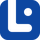# Articles related to "each"

### Each vs. All

These two words are different as their meanings require.

### Each vs. Both

'Both' and 'each' have two different meanings which makes them easy to understand.

### Distributive Pronouns

Distributive pronouns refer to nouns separately rather than collectively in a group. In this lesson, we will learn all about them.

### Quantifiers

Quantifiers or Quantitatives are a type of determiners that are used with nouns. In this lesson, we will discover their uses, rules, and more in detail.

### Each Other

'Each other' is a reciprocal pronoun. In this part, we will go through its uses and grammatical rules in English grammar.

### Each

Each of us has heard the word 'each' but not many of us know its uses. If you want to learn its uses, follow this lesson.

### Each vs. Each Of

Actually, these two words mean the same, but they are used in different situations.

### Universal Pro-forms

Universal pro-forms are special function words or expressions to refer to the total number of something. In this lesson, you will learn more about them.

### Both vs. Each

'Each' and 'both' are easily confused by English learners. They are similar to each other. Follow the article to learn about their similarities and differences.

### Each vs. Every

It is usually correct to use both, ‘each’ or ‘every’, but they have slightly different meanings.

### Distributives

Distributives are determiners that indicate divided groups of people. In this lesson, we will learn uses, grammatical rules, and more.

### Pro-forms

Understanding pronouns enable us to understand pro-forms in English very well. Pro-forms are alternatives that are put in the position of words, phrases, etc.

### Each vs. Every

In this lesson, we're gonna learn about how these two words are different from one another. You know, these grammatical functions are common.

### Indefinite Pronouns

Indefinite pronouns refer to people or things without saying exactly who or what they are. In this lesson, we will learn more about these pronouns.

### Each vs. Either

Actually, either and each are misused a lot. Let us get to know them and clarify their differences.